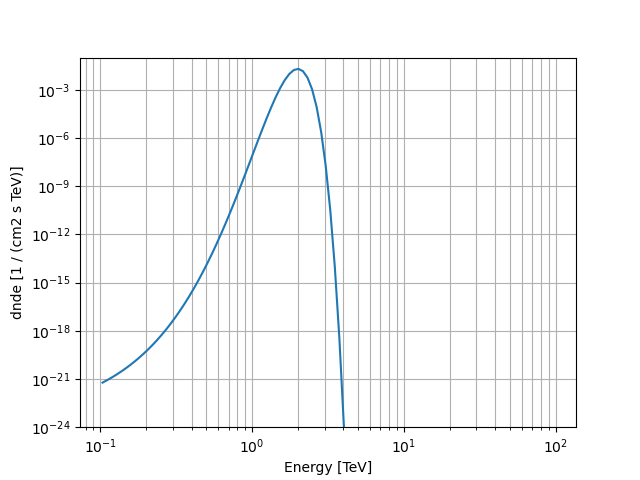# Gaussian spectral model¶

This model parametrises a gaussian spectrum.

It is defined by the following equation:

$\phi(E) = \frac{N_0}{\sigma \sqrt{2\pi}} \exp{ \frac{- \left( E-\bar{E} \right)^2 }{2 \sigma^2} }$

## Example plot¶

Here is an example plot of the model:

from astropy import units as u
import matplotlib.pyplot as plt
from gammapy.modeling.models import GaussianSpectralModel, Models, SkyModel

energy_bounds = [0.1, 100] * u.TeV
model = GaussianSpectralModel(norm="1e-2 cm-2 s-1", mean=2 * u.TeV, sigma=0.2 * u.TeV)
model.plot(energy_bounds)
plt.grid(which="both")
plt.ylim(1e-24, 1e-1)## YAML representation¶

Here is an example YAML file using the model:

model = SkyModel(spectral_model=model, name="gaussian-model")
models = Models([model])

print(models.to_yaml())


Out:

components:
-   name: gaussian-model
type: SkyModel
spectral:
type: GaussianSpectralModel
parameters:
-   name: norm
value: 0.01
unit: cm-2 s-1
-   name: mean
value: 2.0
unit: TeV
-   name: sigma
value: 0.2
unit: TeV


Gallery generated by Sphinx-Gallery